Courses

# Test: The P-Block Elements 2 - From Past 28 Years Questions

## 30 Questions MCQ Test Chemistry Class 12 | Test: The P-Block Elements 2 - From Past 28 Years Questions

Description
This mock test of Test: The P-Block Elements 2 - From Past 28 Years Questions for NEET helps you for every NEET entrance exam. This contains 30 Multiple Choice Questions for NEET Test: The P-Block Elements 2 - From Past 28 Years Questions (mcq) to study with solutions a complete question bank. The solved questions answers in this Test: The P-Block Elements 2 - From Past 28 Years Questions quiz give you a good mix of easy questions and tough questions. NEET students definitely take this Test: The P-Block Elements 2 - From Past 28 Years Questions exercise for a better result in the exam. You can find other Test: The P-Block Elements 2 - From Past 28 Years Questions extra questions, long questions & short questions for NEET on EduRev as well by searching above.
QUESTION: 1

### Which of the following fertilizers has the highest nitrogen percentage ? 

Solution:

Urea (46.6%N). % of N in other compound are : ( NH4)2 SO4 = 21.2%; CaCN2 = 35.0% and NH4 NO3 = 35.0%

QUESTION: 2

### A solution of potassium bromide is treated with each of the following. Which one would liberate bromine ? 

Solution:

The correct answer is option C
Chlorine is more reactive than bromine and it displaces bromine.
If chlorine is added to a solution of potassium bromide, the bromine is replaced by the chlorine forming potassium chloride. Bromine is formed at the same time and can be detected by its colour.
2KBr + Cl2 ​⟶ 2KCl + Br2​

QUESTION: 3

### A certain compound (X) when treated with copper sulphate solution yields a brown precipitate. On adding hypo solution, the precipitate turns white.The compound is

Solution:

KI reacts with CuSO4 solution to produce cuprous iodide (white precipitate) and I2 (which gives brown colour) Iodine reacts with hypo (Na2S2O35H2O) solution.
Decolourisaiton of solution shows the appearance of white precipitate.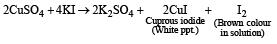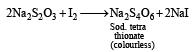QUESTION: 4

Noble gases do not react with other elements because 

Solution:

On account of highly stable ns2 np6 configuration in the valence shell. These elements have no tendency either to lose gain or share electrons with atoms of other elements i.e., their combining capacity or valency is zero. Further all the orbitals in the atoms of these elements are doubly occupied i.e electrons are not available for sharing.

QUESTION: 5

Which of the following statements is false ? 

Solution:

The most abundant rare gas found in the atmosphere is argon and not helium.

QUESTION: 6

HI can be prepared by all the following methods, except

Solution:

HI cannot be prepared by heating iodides with concentrated H2SO4 because HI is a strong reducing agent and reduce H2SO4 to SO2 and get themselves oxidised to iodide.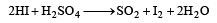QUESTION: 7

Which among the following is paramagnetic ? 

Solution:

ClO2 contain s 7 + 12 i.e. 19 electr ons (valence) which is an odd number, i.e. there is (are) free electron(s). Hence it is paramagnetic in nature.

QUESTION: 8

Which one of the following oxides of chlorine is obtained by passing dry chlorine over silver chlorate at 90°C ? 

Solution:

Pure ClO2 is obtained by passing dry Cl2 over AgClO3 at 90°C.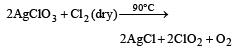QUESTION: 9

The formula for calcium chlorite is 

Solution:

Calcium chlorite is Ca (ClO2)2

QUESTION: 10

Polyanion formation is maximum in 

Solution:

Due to greater tendency for catenation, sulphur shows property of polyanion formation to a greater extent. For example, in polysulphides such as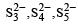QUESTION: 11

The acid which has a peroxy linkage is 

Solution:

Caro’s acid is H2SO5 which contains one S – O – O – H peroxy linkage. It is also known as permonosulphuric acids.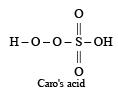QUESTION: 12

Brown ring test is used to detect 

Solution:

Brown ring test is done for confirmation of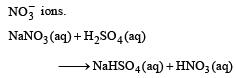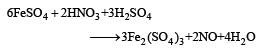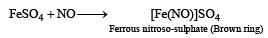QUESTION: 13

About 20 km above the earth, there is an ozone layer. Which one of the following statements about ozone and ozone layer is true? 

Solution:

Ozone layer is beneficial to us, because it stops harmful ultraviolet radiations to reach the earth.

QUESTION: 14

By passing H2S gas in acidified KMnO4 solution, we get 

Solution: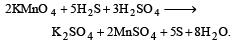Thus in this reaction sulphur (S) is produced.

QUESTION: 15

Regarding F and Cl which of the following statements is/are correct? 
(i) Cl can give up an electron more easily than F
(ii) Cl is a better reducing agent than F
(iii) Cl is smaller in size than F
(iv)F can be oxidized more readily than Cl

Solution: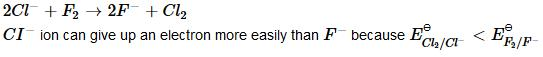QUESTION: 16

Which of the following oxides will be the least acidic?

Solution:

As th e O.N of the central atom of the compounds increases acidic strength of that compound also increases and on moving from top to bottom in groups acidic strength of oxides also decrease due to decreasing electronegativity in groups.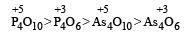QUESTION: 17

Oxidation of thiosulphate by iodine gives 

Solution: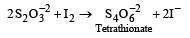QUESTION: 18

Which one is the correct order of the size of iodine species? 

Solution:

We know that positive ion is always smaller and negative ion is always larger than the corresponding atom. Therefore the correct order of the size is I- > I > I+

QUESTION: 19

Which of the following species has the highest dipole moment ? 

Solution:

Order of dipole moment decreases as NH3 > PH3 > AsH3 > SbH3 (Based upon electronegativity)

QUESTION: 20

The structural formula of hypophosphorous acid is

Solution:

We know that empirical formula of hypophosphorus acid is H3PO2. In this only one ionisable hydrogen atom is present i.e. it is monobasic. Therefore option (1) is correct structural formula of it.

QUESTION: 21

A one litre flask is full of brown bromine vapour.The intensity of brown colour of vapour will not decrease appreciably on adding to the flask some

Solution:

Due to absence of reaction between marble and bromine

QUESTION: 22

Repeated use of which one of the following fertilizers would increase the acidity of the soil?

Solution:

Ammonium sulphate is a salt of weak base and strong acid, so it produces acidity. Hence aqueous solution of ammonium sulphate is increases the acidity of soil.

QUESTION: 23

During its reactions, ozone 

Solution:

Since ozone can easily lose oxygen atom (nascent oxygen), it acts as a powerful oxidising agent, and hence reacts with hydrogen atoms.

QUESTION: 24

Which of the following oxy-acids has the maximum number of hydrogens directly attached to phosphorus? 

Solution: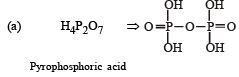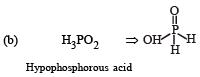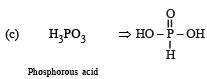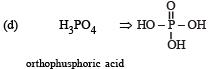QUESTION: 25

Which one of the following arrangements does not truly represent the property indicated against it?

Solution:

The bond energy of F2 < Cl2 due to more repulsion in between non-bonding electrons (2p) of F2 in comparision to non- bonding pair (3p) repulsion in Cl2, the bond energy of F2 < Cl2.
Bond energy (kJ mol–1) :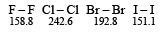QUESTION: 26

Nitrogen forms N2, but phosphorus is converted into P4 from P, the reason is 

Solution:

Nitrogen form N2 (i.e. N ≡ N) but phosphorus form P4, because in P2, pπ — pπ bonding is present which is a weaker bonding.

QUESTION: 27

Which of the following statements is not true ? 

Solution:

F is more electronegative than Cl therefore HF bond is stronger than HCl and hence proton is not given off easily and hence HF is a weakest acid.

QUESTION: 28

Which is the best description of the behaviour of bromine in the reaction given below ?  H2O + Br2 → HOBr + HBr

Solution: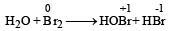Thus here oxidation number of Br increases from 0 to +1 and also decreases from 0 to –1.
Thus  it is oxidised as well as  reduced

QUESTION: 29

The correct order of acid strength is: 

Solution:

As oxidation number of central atom in oxyacid increases strength increases.  Hence the correct order of acidic strength is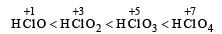QUESTION: 30

Which of the following is the most basic oxide?

Solution:

More the oxidation state of the central atom (metal) more is its acidity. Hence SeO2 (O. S. of Se = +4) is acidic. Further for a given O.S., the basic character of the oxides increases with the increasing size of the central atom.
Thus Al2O3 and Sb2O3 are amphoteric and Bi2O3 is basic.